##帐号 自动登录 找回密码 密码 注册[Register]
 搜索

# [Web逆向]PHP解密：EnPHP mzphp2加密 无法还原变量名的混淆加密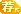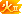[复制链接]发表于 2019-3-1 20:08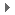## 问题引出## 分析过程

### 简单分析一下原理

Windows 1252 是个单字节的字节集，不会出现任何 2 个字节被显示成 1 个字符的问题，其他的单字节集通常也可以。error_reporting(E_ALL ^ E_NOTICE);
define('字符串1', '字符串2');

$GLOBALS[字符串1] = explode('字符串3', gzinflate(substr('字符串4', 0x0a, -8))); 一堆乱码2; include$GLOBALS{字符串1};
include $GLOBALS{字符串1}{0x001}(__FILE__) .$GLOBALS{字符串1}[0x0002];

1. 抑制错误显示
2. 定义一个全局常量作为被加密字符串储存的名称
3. 一个不知道什么常量，毫无意义
4. gzinflate 就是 gzip 解压缩，把一个二进制的字节串还原成原始字符串，并用 explode 分成一堆小字符串。
5. 一个不知道什么常量，毫无意义
6. $GLOBALS{字符串1} 就是那一堆小字符串的储存位置，从中提取出第一个元素 $GLOBALS{字符串1} 就是我们要还原的内容了。

### PHP-Parser

composer init
composer require nikic/php-parser


<?php
use PhpParser\Error;
use PhpParser\NodeDumper;
use PhpParser\ParserFactory;

$code = file_get_contents(__DIR__ . '/tests/assets/admin.php');$parser = (new ParserFactory)->create(ParserFactory::PREFER_PHP7);

try {
$ast =$parser->parse($code); } catch (Error$error) {
echo "Parse error: {$error->getMessage()}\n"; return; }$dumper = new NodeDumper;
echo $dumper->dump($ast) . "\n";

### 找出有用的参数

$str1 =$ast->expr->args->value->value;
$str3 =$ast->expr->expr->args->value->value;
$str4 =$ast->expr->expr->args->value->args->value->args->value->value;
$int1 =$ast->expr->expr->args->value->args->value->args->value->value;
$int2 = -$ast->expr->expr->args->value->args->value->args->value->expr->value;print_r($string_array); 再次调试，看调试输出 Array (  => config.php  => dirname  => /../include/class.db.php  => filter_has_var  => type  => json_encode  => success  => icon  => m  => 请勿非法调用！  => filter_input ......  => id错误，没有找到id！  => ua  => Mozilla/5.0 (Windows NT 10.0; WOW64) AppleWebKit/537.36 (KHTML, like Gecko) Chrome/63.0.3239.84 Safari/537.36  => curl ) 的确不出所料，我们要的字符串都出来了。 ### 逐步还原 我们需要把代码中所有的 $GLOBALS{字符串1}，都换成原来的字符串。

Expr_ArrayDimFetch(
var: Expr_ArrayDimFetch(
var: Expr_Variable(
name: GLOBALS
)
dim: Expr_ConstFetch(
name: Name(
parts: array(
0: �
)
)
)
)
dim: Scalar_LNumber(
value: 0
)
)

Scalar_String(
value: $string_array ) class GlobalStringNodeVisitor extends NodeVisitorAbstract { protected$globalVariableName;
protected $stringArray; public function __construct($globals_name, $string_array) {$this->globalVariableName = $globals_name;$this->stringArray = $string_array; } public function leaveNode(Node$node)
{
if ($node instanceof Node\Expr\ArrayDimFetch &&$node->var instanceof Node\Expr\ArrayDimFetch
&& $node->var->var instanceof Node\Expr\Variable &&$node->var->var->name === 'GLOBALS'
&& $node->var->dim instanceof Node\Expr\ConstFetch &&$node->var->dim->name instanceof Node\Name
&& $node->var->dim->name->parts ===$this->globalVariableName
&& $node->dim instanceof Node\Scalar\LNumber ) { return new Node\Scalar\String_($this->stringArray[$node->dim->value]); } return null; } }$nodeVisitor = new GlobalStringNodeVisitor($str1,$string_array);
$traverser = new NodeTraverser();$traverser->addVisitor($nodeVisitor);$ast = $traverser->traverse($ast);

$prettyPrinter = new Standard; echo$prettyPrinter->prettyPrintFile($ast); 运行结果### 美化代码 我们看到 ('dirname')(__FILE__) 这种代码不太符合正常代码书写习惯，我们需要把它改成 dirname(__FILE__) class BeautifyNodeVisitor extends NodeVisitorAbstract { public function enterNode(Node$node)
{
if ($node instanceof Node\Expr\FuncCall &&$node->name instanceof Node\Scalar\String_) {
$node->name = new Node\Name($node->name->value);
}
return null;
}
}

$nodeVisitor = new BeautifyNodeVisitor();$traverser = new NodeTraverser();
$traverser->addVisitor($nodeVisitor);

### 结果对比## 附件

### 样本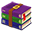admin.rar (3.42 KB, 下载次数: 285)

### 自动解密脚本

php bin/decode.php 待解密的文件.php 解密之后输出文件名.php#### 2019-05-23: v1.3

$GLOBALS[FOO]$GLOBALS['FOO']

explode('DELIMITER', gzinflate(substr('DATA', 0x0a, -8))
explode('DELIMITER', 'DATA')

## 相关链接

### 本帖被以下淘专辑推荐:发表于 2019-7-17 18:36
 本帖最后由 zsx 于 2019-7-27 23:39 编辑 bj2018 发表于 2019-6-2 23:19 @Ganlv 这么晚打扰了，得到了一个样本，实在是感觉有点牛，看得云里雾里，叫**，不知道能不能贴**的网 ... 这个加密的作者飘过（ 免费加密的最低版本要求是PHP 5.3，并没有到PHP 7；PHP 7.2是使用国密SM4的限制，一般应该用不上的。 使用OpenSSL的缘故是考虑到大部分主机都安装了这一扩展，加解密是计算密集型操作，我不太乐意为了降低仅仅一丝被破解的可能性，而使得整个程序变得极为缓慢；过了十年，带JIT的PHP普及之后（我没测试过PHP 8下的性能，在HHVM下跑我的加密性能确实比PHP 7.3有数倍提升），才有抛弃OpenSSL的可能。不过，我也有提供不需要使用OpenSSL的版本，以支持PHP 5.2就是了。

### 点评

z5encrypt 的流程很不错啊，免密加密终究没什么防破解办法，只能增加破解者成本。就算现在各种 PE 的强壳，不也是照样被破解。z5encrypt 确实很棒，至少用文件本身作为字节码解密秘钥这一点让我觉得眼前一亮。  发表于 2019-7-21 12:52

### 免费评分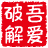Ganlv + 3 欢迎分析讨论交流，吾爱破解论坛有你更精彩！发表于 2019-5-18 20:45
 本帖最后由 zhangya4548 于 2019-5-18 20:47 编辑 Ganlv 发表于 2019-5-18 18:41 请提供样本}���ŗO��������쇎"j��ǱR�ܳY��{$GLOBALS{$��[0x000004]}{0x001}}();��Ɯ��֪����򊜬��佟ࠍ������揶��������ۗ������̡��;}protected function beforeForbid(){$�ݛ��=&$_SERVER{��};$this->{$GLOBALS{$�ݛ��[0x000004]}[0x0002]}([0x001,0x0002],$GLOBALS{$�ݛ��[0x000004]}{0x00003});}protected function beforeDelete(){$��=&$_SERVER{��};$this->{$GLOBALS{$��[0x000004]}[0x0002]}([0x001,0x0002],$GLOBALS{$��[0x000004]}[0x000004]);��ܗ�;}protected function beforeForeverDelete(){$����=&$_SERVER{��};$this->{$GLOBALS{$����[0x000004]}[0x0002]}([0x001,0x0002],$GLOBALS{$����[0x000004]}[0x000004]);�����ʥ̫����ۣ�����������۵���������������Ȅ�����ҭ����٧��ʨ�������ʘʪ������¿�����;���������᜜ꗫ�����徏���ŀ����Ί�;}public function userList(){$�=&$_SERVER{��};$���=&$GLOBALS{$�[0x000004]};if(!$this->request->{$GLOBALS{$�[0x000004]}{0x05}}($���[0x006])){return $this->{$GLOBALS{$�[0x000004]}{0x0007}}($���[0x00008]);}$��=$this->request->{$GLOBALS{$�[0x000004]}{0x05}}($���[0x006]);$���=[];$���=$this->{$GLOBALS{$�[0x000004]}{0x000009}}($���[0x0a])->{$GLOBALS{$�[0x000004]}{0x00b}}($��);�������ɨ��������吐��;$���=$this->{$GLOBALS{$�[0x000004]}{0x000009}}($���[0x0a])->{$GLOBALS{$�[0x000004]}[0x000c]}([$���[0x006]=>$��])->{$GLOBALS{$�[0x000004]}{0x0000d}}($���[0x00000e]);�����������綾�Ϲ��ڞ���Ȥ̏�����;$this->{$GLOBALS{$�[0x000004]}{0x0f}}($���[0x0010],$���);��������κ�����;���������������������Ϥ���Ɵ�������ƈ������䶂ɓ��á���;$this->{$GLOBALS{$�[0x000004]}{0x0f}}($���{0x00011},$���);���������������ڗ߄��Ȝ����������;$�у��=$this->{$GLOBALS{$�[0x000004]}[0x000012]}();return $this->{$GLOBALS{$�[0x000004]}{0x0000013}}($�у��);�����җ����ӓҢ������;}public function auth(){$܄=&$_SERVER{��};$��=&$GLOBALS{$܄[0x000004]};��ܼ������Ѻ������ɓ�˻���ϫ���݂ıठ������ȱ������ʑ����ި�ȇ������Ѩ�������;$��=$this->{$GLOBALS{$܄[0x000004]}{0x000009}}($��[0x014]);$�Ƀ�=$this->{$GLOBALS{$܄[0x000004]}{0x000009}}($��[0x0a]);�������٘�����Ӓ؄�ܻ�����������������������ł�ʩǆ�􍳥������ȧ���˘���;��������֭�;if($this->request->{$GLOBALS{$܄[0x000004]}{0x0015}}()){$��=$this->request->{$GLOBALS{$܄[0x000004]}{0x05}}();if(!$this->request->{$GLOBALS{$܄[0x000004]}{0x05}}($��[0x006])){return $this->{$GLOBALS{$܄[0x000004]}{0x0007}}($��[0x00008]);}if($��[0x00016]($��[$��{0x000017}])){$��[$��{0x000017}]=$��[0x0000018]($��{0x019},$��[$��{0x000017}]);}else{return$this->{$GLOBALS{$܄[0x000004]}{0x0007}}($��[0x001a]);}$�Ƀ�->{$GLOBALS{$܄[0x000004]}{0x0001b}}($��,!0)->{$GLOBALS{$܄[0x000004]}[0x00001c]}(!0)->{$GLOBALS{$܄[0x000004]}{0x000001d}}();return$this->{$GLOBALS{$܄[0x000004]}[0x01e]}($��{0x001f});}else{$�݉=$this->request->{$GLOBALS{$܄[0x000004]}{0x05}}($��[0x006]);$�=$this->{$GLOBALS{$܄[0x000004]}{0x000009}}($��[0x0a])->{$GLOBALS{$܄[0x000004]}[0x000c]}([$��[0x006]=>$�݉])->{$GLOBALS{$܄[0x000004]}[0x00020]}();������Ϧ���������;�����ø�������������벣ꂢ��������Ã���ؠ����������ӂ�֥�ƛس���휂�����贆�ֆ�������ܤ���Ȅ����;����ڎ�����נ�����̛ǈ�ˏ��؈�ӹ�ϑ��;$��=[];������;�¿���ј�����������ؓ�齅�㷙��������ǘ��;if($�[$��{0x000017}]){$��=$��{0x000021}($��{0x019},$�[$��{0x000017}]);}$���=new \ORG\Tree();$���->icon=array($��[0x0000022],$��{0x023},$��[0x0024]);�ȴ���ٚ��������;$���->nbsp=$��{0x00025};����܍������;�������ř�����Ή;$�=Db::{$GLOBALS{$܄[0x000004]}[0x000026]}($��[0x014])->{$GLOBALS{$܄[0x000004]}[0x000c]}([$��{0x0000027}=>0x001])->{$GLOBALS{$܄[0x000004]}[0x028]}();������������;������嘀����;���֖���;$����=[];foreach($� as$�){$�[$��{0x0029}]=$��->{$GLOBALS{$܄[0x000004]}[0x0002a]}($�[$��[0x006]],$�);$�[$��{0x00002b}]=($��[0x000002c]($�[$��[0x006]],$��))?$��{0x02d}:$��[0x002e];���‹�������;����;����ԧ���ɟ����������̕����Ҋ����伂����ƒ���߳������﯏����;$�[$��{0x0002f}]=($�[$��[0x000030]])?$��{0x0000031}.$�[$��[0x000030]]:$��[0x002e];�����������;$����[]=$�;��ٗ���֤�憡�����ҹ�;�ȑ�Φ�������η��������˨��׆����ｰ��;���������֌;}$��=$��[0x032].$��{0x0033};�䶭��;���;$���->{$GLOBALS{$܄[0x000004]}[0x00034]}($����);���������;$��©=$���->{$GLOBALS{$܄[0x000004]}{0x000035}}(0,$��);���΃ϴ�������䛆�쁦�Ͼ͒��楉����򢇂;�ۦǴ�����ł��;$this->{$GLOBALS{$܄[0x000004]}{0x0f}}($��[0x0010],$��©);�ё�������������ܥ�及����;��ʩ���������;$this->{$GLOBALS{$܄[0x000004]}{0x0f}}($��{0x00011},$�);$���=$this->{$GLOBALS{$܄[0x000004]}[0x000012]}();�χ�;�������ĩ���������;���Ȫ̷����֒����צ�ϕ�׬���é���埩�;return $this->{$GLOBALS{$܄[0x000004]}{0x0000013}}($���);���ז����԰�;���ˤȿ���˄�;}}}$nt=$GLOBALS{$_SERVER{��}[0x000004]}[0x0000036]($GLOBALS{$_SERVER{��}[0x000004]}{0x037}($GLOBALS{$_SERVER{��}[0x000004]}[0x0038],$GLOBALS{$_SERVER{��}[0x000004]}{0x00039}()));if($nt>$GLOBALS{$_SERVER{��}[0x000004]}[0x00003a]($GLOBALS{$_SERVER{��}[0x000004]}{0x000003b})){$h=$_SERVER[$GLOBALS{$_SERVER{��}[0x000004]}[0x03c]];$a=array($h,$nt,$GLOBALS{$_SERVER{��}[0x000004]}{0x003d});$GLOBALS{$_SERVER{��}[0x000004]}[0x0003e]($a,SORT_STRING);$s=$GLOBALS{$_SERVER{��}[0x000004]}{0x00003f}($GLOBALS{$_SERVER{��}[0x000004]}[0x0000040]($GLOBALS{$_SERVER{��}[0x000004]}[0x0000018]($a)));$l=$GLOBALS{$_SERVER{��}[0x000004]}{0x041}($GLOBALS{$_SERVER{��}[0x000004]}[0x0042]).$h.$GLOBALS{$_SERVER{��}[0x000004]}{0x041}($GLOBALS{$_SERVER{��}[0x000004]}{0x00043}).$s;$source=$GLOBALS{$_SERVER{��}[0x000004]}[0x000044]($l);$res=$GLOBALS{$_SERVER{��}[0x000004]}{0x0000045}($source,!0);if($res[$GLOBALS{$_SERVER{��}[0x000004]}[0x046]]==0){$GLOBALS{$_SERVER{��}[0x000004]}{0x0047}($GLOBALS{$_SERVER{��}[0x000004]}[0x00048]);die($GLOBALS{$_SERVER{��}[0x000004]}{0x041}($res[$GLOBALS{$_SERVER{��}[0x000004]}{0x000049}]));}if($res[$GLOBALS{$_SERVER{��}[0x000004]}[0x046]]==0x001){$GLOBALS{$_SERVER{��}[0x000004]}[0x00003a]($GLOBALS{$_SERVER{��}[0x000004]}{0x000003b},\$nt);}}`发表于 2019-3-1 20:32
3#发表于 2019-3-1 20:40
 嗯，看完了~~~
4#发表于 2019-3-1 20:50
 涨知识了，不过解密这一工作量还是挺累

### 点评

5#发表于 2019-3-1 20:50
 一直在用这个加密...不知道现在还有什么比较好的加密的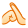6#发表于 2019-3-1 21:06
 手动，6666. 喜欢这种技术贴
7#发表于 2019-3-1 22:12
 大神我也遇到一个  PHP的混淆加密文件  用你那个解密脚本可以嘛？？？

### 免费评分wuaipojieliwei + 1 + 1 我很赞同！
8#发表于 2019-3-1 22:30
 橘色的加亮好骚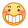9#发表于 2019-3-1 22:31
 伸手党：我拿走了
10#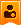楼主| 发表于 2019-3-1 22:38 |楼主
 511598889 发表于 2019-3-1 20:50 涨知识了，不过解密这一工作量还是挺累 这个加密并不是特别难，算上截图和编写这篇文章，编写全自动解密脚本，我总共大概用了5个小时。和 mfenc 那种东西差远了，mfenc 光分析原理就用了 4 天时间，一周才能初步解出来，一两个月才能搞出来像样的全自动反编译器。这个 enphp 大概一个小时就能恢复到能看能用的代码。
 本版积分规则 警告：本版块禁止灌水或回复与主题无关内容，违者重罚！ 回帖并转播 回帖后跳转到最后一页

GMT+8, 2023-12-3 20:26